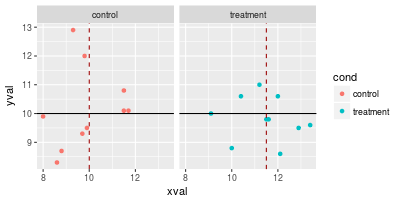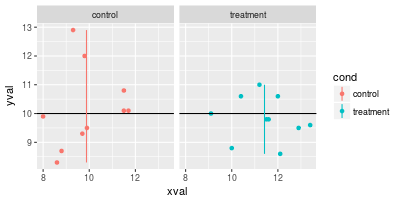## Problem

You want to do add lines to a plot.

## Solution

### With one continuous and one categorical axis

``````# Some sample data
cond result
control     10
treatment   11.5
')

library(ggplot2)
``````

#### Lines that go all the way across

These use `geom_hline` because the y-axis is the continuous one, but it is also possible to use `geom_vline` (with `xintercept`) if the x-axis is continuous.

``````# Basic bar plot
bp <- ggplot(dat, aes(x=cond, y=result)) +
geom_bar(position=position_dodge(), stat="identity")
bp

bp + geom_hline(aes(yintercept=12))

# Make the line red and dashed
bp + geom_hline(aes(yintercept=12), colour="#990000", linetype="dashed")
``````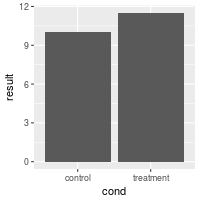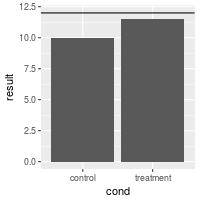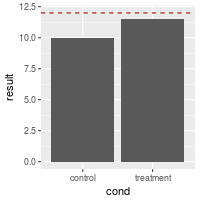#### Separate lines for each categorical value

To make separate lines for each bar, use `geom_errorbar`. The error bars have no height – `ymin`=`ymax`. It also seems necessary to specify `y` for some reason, even though it doesn’t do anything.

``````# Draw separate hlines for each bar. First add another column to dat
dat\$hline <- c(9,12)
dat
#>        cond result hline
#> 1   control   10.0     9
#> 2 treatment   11.5    12

# Need to re-specify bp, because the data has changed
bp <- ggplot(dat, aes(x=cond, y=result)) +
geom_bar(position=position_dodge(), stat="identity")

# Draw with separate lines for each bar
bp + geom_errorbar(aes(ymax=hline, ymin=hline), colour="#AA0000")

# Make the lines narrower
bp + geom_errorbar(width=0.5, aes(ymax=hline, ymin=hline), colour="#AA0000")

# Can get the same result, even if we get the hline values from a second data frame
# Define data frame with hline
dat_hlines <- data.frame(cond=c("control","treatment"), hline=c(9,12))
dat_hlines
#>        cond hline
#> 1   control     9
#> 2 treatment    12

# The bars are from dat, but the lines are from dat_hlines
bp + geom_errorbar(data=dat_hlines, aes(y=NULL, ymax=hline, ymin=hline), colour="#AA0000")
#> Warning: Ignoring unknown aesthetics: y
``````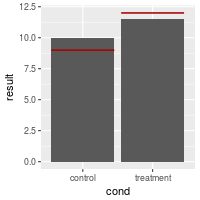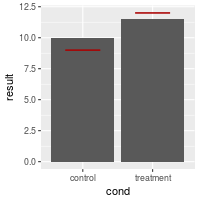#### Lines over grouped bars

It is possible to add lines over grouped bars. In this example, there are actually four lines (one for each entry for `hline`), but it looks like two, because they are drawn on top of each other. I don’t think it’s possible to avoid this, but it doesn’t cause any problems.

``````dat <- read.table(header=TRUE, text='
cond group result hline
control     A     10     9
treatment     A   11.5    12
control     B     12     9
treatment     B     14    12
')
dat
#>        cond group result hline
#> 1   control     A   10.0     9
#> 2 treatment     A   11.5    12
#> 3   control     B   12.0     9
#> 4 treatment     B   14.0    12

# Define basic bar plot
bp <- ggplot(dat, aes(x=cond, y=result, fill=group)) +
geom_bar(position=position_dodge(), stat="identity")
bp

# The error bars get plotted over one another -- there are four but it looks
# like two
bp + geom_errorbar(aes(ymax=hline, ymin=hline), linetype="dashed")
``````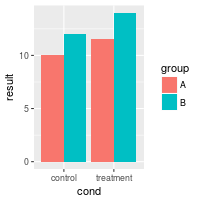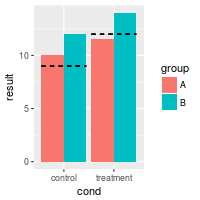#### Lines over individual grouped bars

It is also possible to have lines over each individual bar, even when grouped.

``````dat <- read.table(header=TRUE, text='
cond group result hline
control     A     10    11
treatment     A   11.5    12
control     B     12  12.5
treatment     B     14    15
')

# Define basic bar plot
bp <- ggplot(dat, aes(x=cond, y=result, fill=group)) +
geom_bar(position=position_dodge(), stat="identity")
bp

bp + geom_errorbar(aes(ymax=hline, ymin=hline), linetype="dashed",
position=position_dodge())
``````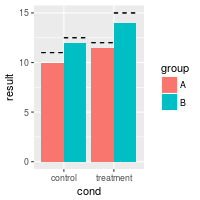### With two continuous axes

Sample data used here:

``````dat <- read.table(header=TRUE, text='
cond xval yval
control 11.5 10.8
control  9.3 12.9
control  8.0  9.9
control 11.5 10.1
control  8.6  8.3
control  9.9  9.5
control  8.8  8.7
control 11.7 10.1
control  9.7  9.3
control  9.8 12.0
treatment 10.4 10.6
treatment 12.1  8.6
treatment 11.2 11.0
treatment 10.0  8.8
treatment 12.9  9.5
treatment  9.1 10.0
treatment 13.4  9.6
treatment 11.6  9.8
treatment 11.5  9.8
treatment 12.0 10.6
')

library(ggplot2)
``````

#### Basic lines

``````# The basic scatterplot
sp <- ggplot(dat, aes(x=xval, y=yval, colour=cond)) + geom_point()

sp + geom_hline(aes(yintercept=10))

# Add a red dashed vertical line
sp + geom_hline(aes(yintercept=10)) +
geom_vline(aes(xintercept=11.5), colour="#BB0000", linetype="dashed")
``````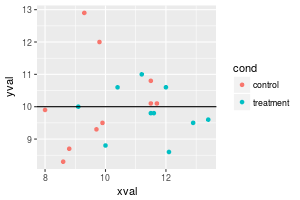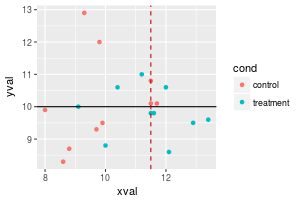#### Drawing lines for the mean

It is also possible to compute a mean value for each subset of data, grouped by some variable. The group means would have to be computed and stored in a separate data frame, and the easiest way to do this is to use the dplyr package. Note that the y range of the line is determined by the data.

``````library(dplyr)
lines <- dat %>%
group_by(cond) %>%
summarise(
x = mean(xval),
ymin = min(yval),
ymax = max(yval)
)

# Add colored lines for the mean xval of each group
sp + geom_hline(aes(yintercept=10)) +
geom_linerange(aes(x=x, y=NULL, ymin=ymin, ymax=ymax), data=lines)
#> Warning: Ignoring unknown aesthetics: y
``````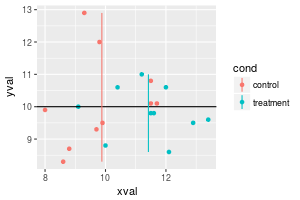#### Using lines with facets

Normally, if you add a line, it will appear in all facets.

``````# Facet, based on cond
spf <- sp + facet_grid(. ~ cond)
spf

# Draw a horizontal line in all of the facets at the same value
spf + geom_hline(aes(yintercept=10))
``````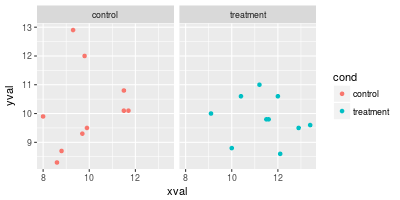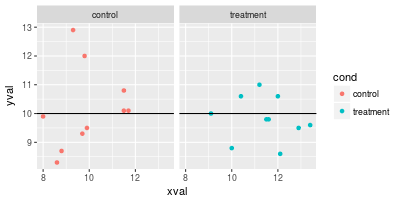If you want the different lines to appear in the different facets, there are two options. One is to create a new data frame with the desired values for the lines. Another option (with more limited control) is to use `stat` and `xintercept` in `geom_line()`.

``````dat_vlines <- data.frame(cond=levels(dat\$cond), xval=c(10,11.5))
dat_vlines
#>        cond xval
#> 1   control 10.0
#> 2 treatment 11.5

spf + geom_hline(aes(yintercept=10)) +
geom_vline(aes(xintercept=xval), data=dat_vlines,
colour="#990000", linetype="dashed")

spf + geom_hline(aes(yintercept=10)) +
geom_linerange(aes(x=x, y=NULL, ymin=ymin, ymax=ymax), data=lines)
#> Warning: Ignoring unknown aesthetics: y
``````